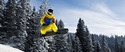## Expressions

Type of Unit: Concept

# Prior Knowledge

Students should be able to:

• Write and evaluate simple expressions that record calculations with numbers.
• Use parentheses, brackets, or braces in numerical expressions and evaluate expressions with these symbols.
• Interpret numerical expressions without evaluating them.

# Lesson Flow

Students learn to write and evaluate numerical expressions involving the four basic arithmetic operations and whole-number exponents. In specific contexts, they create and interpret numerical expressions and evaluate them. Then students move on to algebraic expressions, in which letters stand for numbers. In specific contexts, students simplify algebraic expressions and evaluate them for given values of the variables. Students learn about and use the vocabulary of algebraic expressions. Then they identify equivalent expressions and apply properties of operations, such as the distributive property, to generate equivalent expressions. Finally, students use geometric models to explore greatest common factors and least common multiples.

Subject:
Mathematics, Algebra
Level:
Middle School
6
Tags:
Expresssions, Expressions, Algebraic Expressions, Numerical Expressions, Order of Operations, Greatest Common Factor, Exponents, Concepts, Rectangles, Coefficient, Variables, Distributive Property, Word Problems, Terminology, Math Tricks, Models, 6th Grade Mathematics

Calculators with parentheses buttons (optional, one for each student) (lesson 2)

Unit 4 Expressions
•Lesson 1

Math Tricks
••••••••••Lesson 11

Peer Review
•